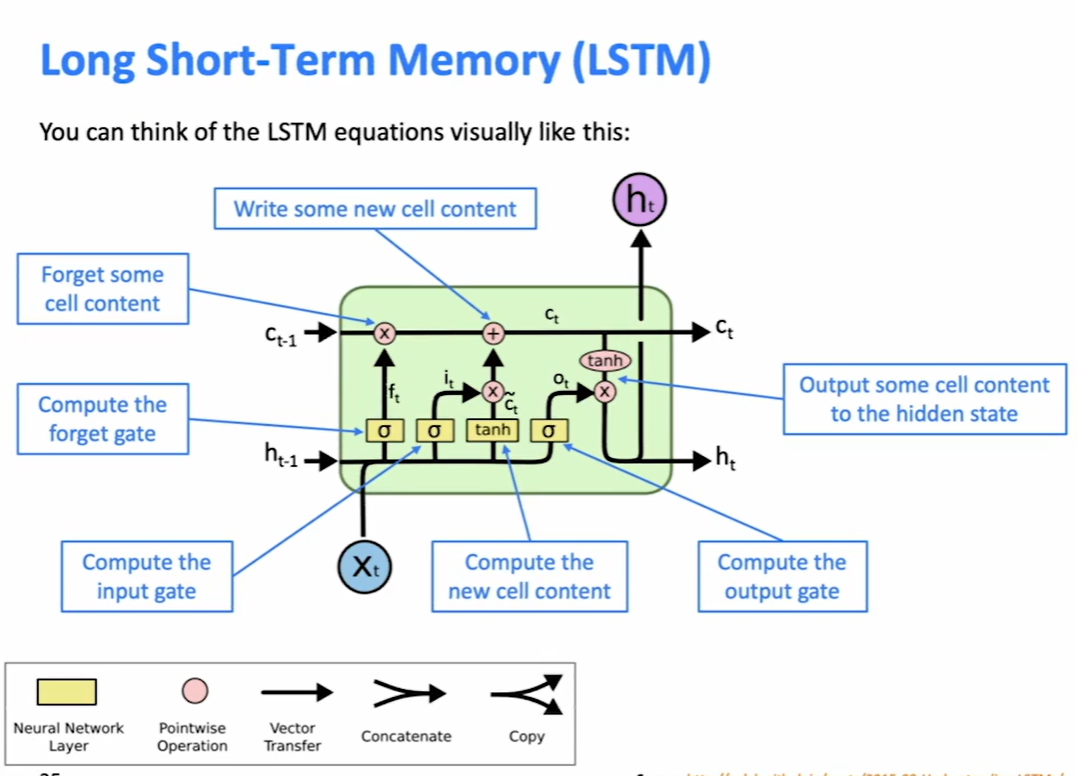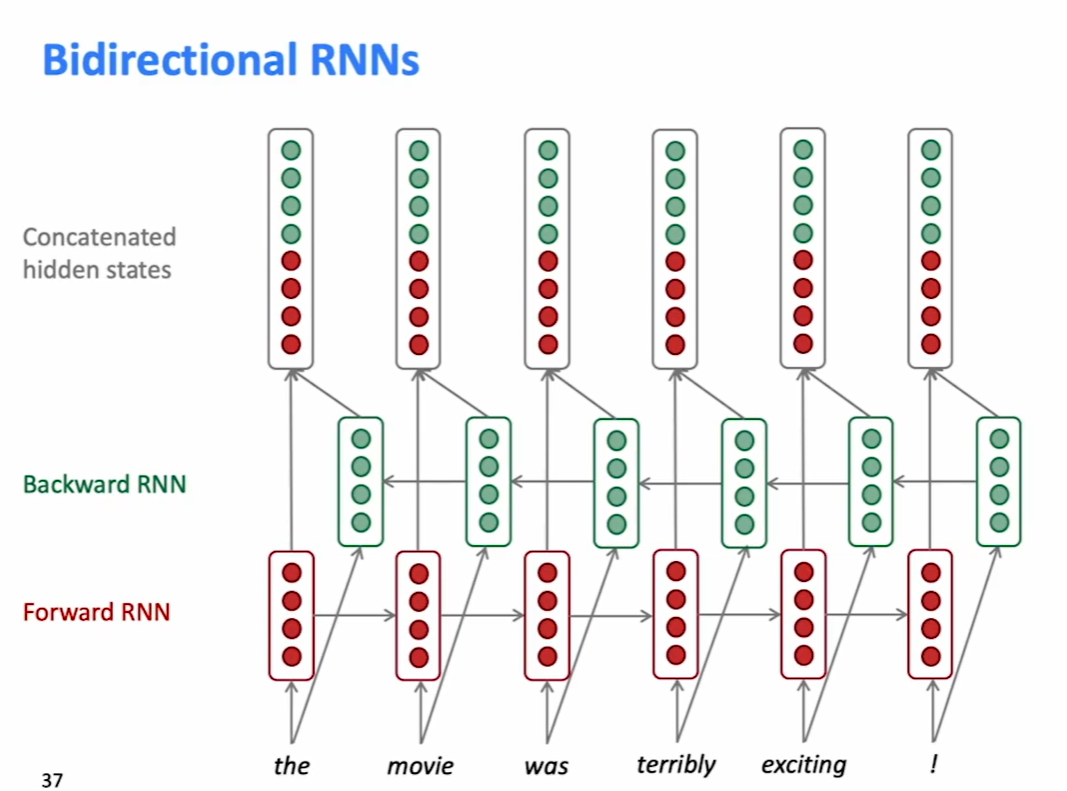# Course 7 - Vanishing Gradients, Fancy RNN

Gradient can be viewed as a measure of the effect of the past on the future

• There is no dependency between step $t$ and $t+n$ in the data
• We have wrong parameters to capture the true dependency between $t$ and $t+n$

## Effect of vanishing gradient on RNN-LM

LM task - unable to predict similar long-distance dependencies

Syntactic recency: The writer of the books is

Sequential recency: The writer of books are

Due to vanishing gradient, RNN-LMs are better at learning from sequential recency than syntactic

## Why is exploding gradient a problem?

Algorithm 1: Pseudo-code for norm clipping

$\hat{g} \leftarrow \frac{\partial \epsilon}{\partial \theta}$

if $||\hat{g}|| \geq threshold$ then

​ $\hat{g} \leftarrow \frac{threshold}{||\hat{g}||} \hat{g}$

end if

## Long Short-Term Memory(LSTM)

On step $t$, there is a hidden state $h^{(t)}$ and a cell state $c^{(t)}$

• Both are vectors length $n$
• The cell stores long-term information
• The LSTM can erase, write and read information from the cell

The selection is controlled by 3 corresponding gates

• vector length $n$
• each element of the gates can be open(1), closed(0), or in between
• dynamic: their value is computed based on the current context

We have a sequence of input $x^{(t)}$, and we will compute a sequence of hidden states $h^{(t)}$ and cell states $c^{(t)}$. On timestep $t$:

Forget gate - controls what is kept vs forgotten, from previous cell state

Input gate - controls what parts of the new cell content are written to cell

Output gate - controls what parts of cell are output to hidden state

New cell content - this is the new content to be written to the cell

Cell state - erase(“forget”) some content from last cell state, and write(“input”) some new cell content

Hidden state: read(“output”) some content from the cell## Gated Recurrent Units(GRU)

a simpler alternative to the LSTM

On each timestep $t$ we have input $x^{(t)}$ and hidden state $h^{(t)}$ (no cell state)

Update gate - controls what parts of hidden state are updated vs preserved

Reset gate - controls what parts of previous hidden state are used to compute new content

New hidden state content - reset gate selects useful parts of prev hidden state. Use this and current input to compute new hidden content.

Hidden state - update gate simultaneously controls what is kept from previous hidden state, and what is updated to new hidden state content

## LSTM vs GRU

LSTM is a good default choice.

Rule of thumb: start with LSTM, but switch to GRU if you want something more efficient

e.g.: Residual connections, “ResNet”. Skip-connections. The identity connection preserves information by default.

e.g.: Dense connections, “DenseNet”.

e.g.:Highway connections, “HighwayNet”.

## Bidirectional RNNs